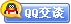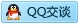##用户名 Email 自动登录 找回密码 密码 会员注册
 VIP会员，3年作业免费下 ！ 奥鹏作业，奥鹏毕业论文检测 新手作业下载教程，充值问题 没有找到答案，请在此处留言！ 2020年04月最新全国统考资料 投诉建议，加盟合作！奥鹏课程积分软件(ver:3.1)

# 2014年秋季学期《C语言》在线考试(适用于2014年12月份考试)答案辅导资料发表于 2015-1-8 14:22:41 | 显示全部楼层 |阅读模式谋学网(www.mouxue.com)是国内最专业的奥鹏作业答案，奥鹏离线作业答案及奥鹏毕业论文辅导型网站，主要提供奥鹏中医大、大工、东财、北语、北航、川大、南开等奥鹏作业答案辅导，致力打造中国最专业的远程教育辅导社区。    2014年秋季学期《C语言》在线考试(适用于2014年12月份考试)        单选题 其他题 简答题 一、单选题（共 10 道试题，共 20 分。） 1.  计算表达式(x=4*5,x*5),x+25的值是 A. 20 B. 125 C. 100 D. 45 -----------------选择：D       2.  有如下定义：long m；char c；float x；double y；则表达式c+m*x/y的值的类型是 A. long B. char C. float D. double -----------------选择：D       3.  有以下函数定义： void fun( int n , double x ) { …… } 若以下选项中的变量都已正确定义并赋值，则对函数fun的正确调用语句是 A. fun( int y , double m ) B. k=fun( 10 , 12.5 ) C. fun( 10 , 12.5 ) D. void fun( 10 ,12.5 )； -----------------选择：C       4.  以下能正确定义数组并正确赋初值的语句是 A. int N=5,b[N][N]; B. int a={{1},{3}}; C. int c[]= {{1,2},{3,4}}; D. int d={{1,2},{34}}; -----------------选择：D       5.  如有以下变量定义：int a=5,b=6,c=12;那么下列表达式结果为真的是 A. a-b B. a>b C. b/c D. c%b -----------------选择：A       6.  在C语言中，下列字符常量中合法的是 A. ′\084′ B. ′\x43′ C. ′ab′ D. ”\0” -----------------选择：       7.  用数组名作为函数调用时的实参，则实际传递给形参的是 A. 数组的第一个元素值 B. 数组中全部元素值 C. 数组的首地址 D. 数组的元素个数 -----------------选择：      8.  执行int j,i=1; j=-i++; 后j的值是 A. -1 B. -2 C. 1 D. 2 -----------------选择：       9.  假设已定义 char c= "test"； int i； 则下面的输出函数调用中错误的是 A. printf("%s",c)； B. for(i=0；i<8；i++) printf("%c",c)； C. puts(c) D. for(i=0；i<8；i++) puts(c)； -----------------选择：       10.  若i为整型变量，则以下循环的次数是for(i=2;i==0;)printf(“%d”,i--); A. 无限次 B. 0次 C. 1次 D. 2次 -----------------选择：          二、其他题（共 10 道试题，共 50 分。）V 1.   读程序写出程序执行结果 #include int f(int b[], int m,int n)     { int i,s=0;       for(i=m;i void main() { int x,y;     for(y=1,x=1; y<=20;y++)     {  if(x>=8) break;        if(x%2==1){ x+=5;  continue ; }        x-=3;     }    printf("x=%d, y=%d\n",x,y); }       满分：5  分 3.   读程序写出程序执行结果 #include void main() { char  x[]="language"; char *ptr=x; while(*ptr)   {  printf("%c\n",*ptr-32);      ptr++;   } }       满分：5  分 4.   读程序写出程序执行结果 #include int d=1 ; int fun(int p) { static int d=3 ;         d+=p;         printf("%3d" , d) ;        return(d) ; } void main( ) { printf("%3d\n" , fun(2+fun(d))) ; }       满分：5  分 5.   读程序写出程序执行结果 #include void main() {  int i,j,m=0;    for(i=1;i<=10;i+=3)       for(j=3;j<10;j+=4)         m++;    printf(“m=%d\n”,m);   }       满分：5  分 6.   读程序写出程序执行结果 #include void main() { int x=26587,x1,r,sum=0; x1=x; while(x>0) { r=x%10; sum+=r; x/=10; } printf( "Sum of the digits in %d is %d", x1,sum); }       满分：5  分 7.   读程序写出程序执行结果 #include void main()     { int i;      for (i=0; i<3; i++)      switch (i)       { case 1: printf("%d ", i);         case 2: printf("%d ", i);         default : printf("%d ", i);   } }       满分：5  分 8.   读程序写出程序执行结果 #include void main() {  int  n= -5 ; while(++n) printf("%3d", ++n) ; }            满分：5  分 9.   读程序写出程序执行结果 #include void main() {  int x[]={1,2,3};    int s,i,*p=x;    s=1;    for(i=0;i<3;i++)       s*=*(p+i);    printf(“%d\n”,s); }       满分：5  分 10.   读程序写出程序执行结果 #include void main()      { int a,*p,*s,i;       for(i=0;i<10;i++)  scanf(%d”,a+i);       for(p=a,s=a;p-a<10;p++)  if(*p>*s)s=p;        printf(“max=%d,index=%d\n”,*s, s-a); } 简述上列程序完成的功能：       满分：5  分 三、简答题（共 3 道试题，共 30 分。）V 1.   编程：计算 sum=1+1/2!+1/3!+……+1/10!, 并输出结果。（备注：建议学员在做编程序题目时，事先在记事本或word文档中编写，然后拷贝至答题区域即可。）       满分：10  分 2.   编程题 备注：建议学员在做编程序题目时，事先在记事本或word文档中编写，然后拷贝至答题区域即可。       满分：10  分 3.  编程：任意输入100个整数，分别统计其中的正数、负数和零的个数。（备注：建议学员在做编程序题目时，事先在记事本或word文档中编写，然后拷贝至答题区域即可。）       满分：10  分 谋学网(www.mouxue.com)是国内最专业的奥鹏作业答案，奥鹏离线作业答案及奥鹏毕业论文辅导型网站，主要提供奥鹏中医大、大工、东财、北语、北航、川大、南开等奥鹏作业答案辅导，致力打造中国最专业的远程教育辅导社区。

### 本帖子中包含更多资源

x
 本版积分规则 回帖并转播 回帖后跳转到最后一页客服一客服二客服三客服四微信客服扫一扫